PSA785V - Grade 8 Mathematics Practice Test 2016

The graph below shows a relationship between x and y.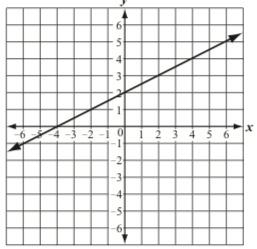Which of the following equations best represents this relationship?

Select one:

What is the value of the following expression?

8-3√16

Select one:

During the first year of operation, a company produced 8.4 X 109 reams of paper. During the second year, the company produced 5.6 times the number of reams of paper that it produced during the first year.

Which expression represents the number of reams of paper the company produced during the second year?

Select one:

What are the solutions to the system of equations below?

3y=x-2

y=-2x+4

Select one:

Quadrilateral JKLM is shown on the coordinate grid below.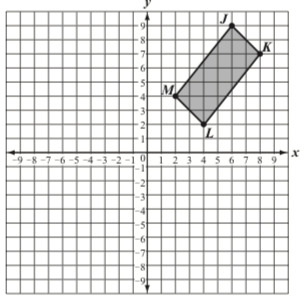Quadrilateral JKLM will be reflected over the x-axis to create its image, quadrilateral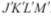. What will be the x-coordinate of the vertex Kl?

Select one:

What is the decimal equivalent of 5/6?

What value of x makes the equation below true?

95•97=9x

x=____

Type your answer below as a number (example: 5, 3.1, 4 1/2, or 3/2):

An equation is incorrectly solved below.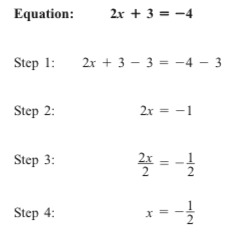What is the first step that shows an error in the solution to the equation?

Select one:

Line and line q are shown on the coordinate grid below.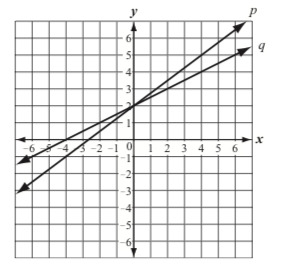Which of the following statements best describes the lines?

Select one:

Which of the following is closest to 0.0432?

Select one:
Part A)

Each of the four functions below shows a relationship between x and y.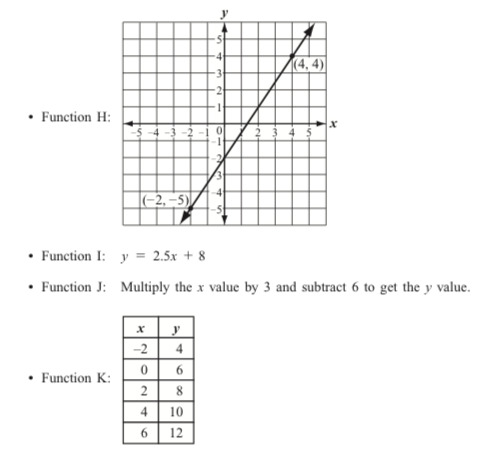What is the slope of the line that represents function H? Show or explain how you got your answer.

Part B)

Write an equation in terms of x and y to represent the graph of Function J.

Part C)

What is the y-intercept of function K? Show or explain how you got your answer.

Part D)

List the four functions in order from the function with the least rate of change to the function with the greatest rate of change.

What is the slope of the line represented by the equation below?

y=(1/3)x+4

Select one:

Lines m, n, and l are parallel. Line w intersects each line as shown in the diagram below.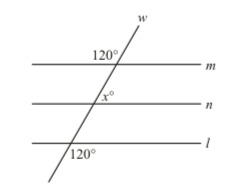Based on the angle measures in the diagram, what is the value of x?

Select one: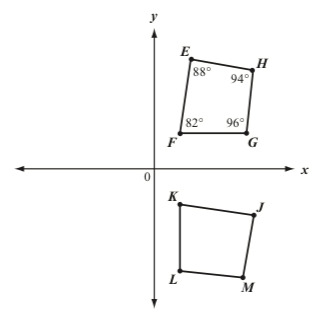What is the measure of angle J?

Select one:

Line segment ST is shown on the coordinate grid below.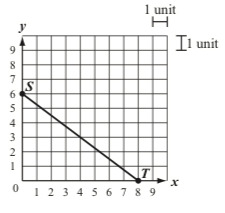What is the length, in units, of line segment ST?

Select one:

Figures Q and R are shown on the coordinate grid below.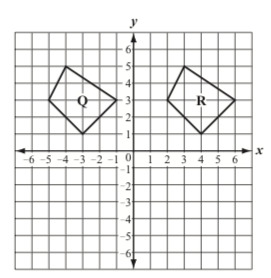Which of the following transformations when performed on Figure Q, will result in Figure R?

Select one:

A jar of peanut butter is in the shape of a cylinder. The jar has an inside radius of 4 centimeters. It is filled with peanut butter to an inside height of 12 centimeters.

What is the total number of cubic centimeters of peanut butter in the jar? (Use 3.14 for pi)

Select one:

A right triangle and some of its measurements are shown below.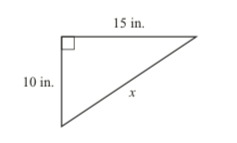What is x, the length to the nearest inch of the third side of the triangle?

Type your answer below as a number (example: 5, 3.1, 4 1/2, or 3/2):

Mia paddled her canoe from the shore of a lake to an island. She stopped on the island to eat lunch, and then paddled her canoe back to the shore. The graph below shows Mia's distance, in kilometers, from the shore over time.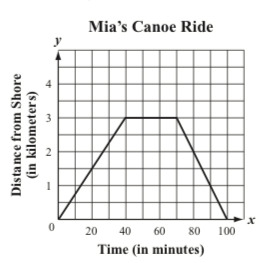Based on the graph, which of the following statements is true?

Select one:

A 9-foot flagpole cast a shadow 6 feet long, and at the same time, a nearby tree cast a shadow 14 feet long, as shown below.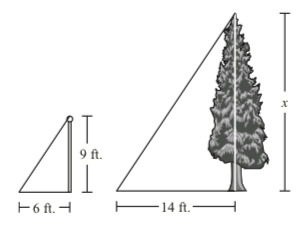What is x, the height of the tree?

Select one:
Part A)

An athletic director wants to add either tennis or gymnastics to the physical education program at her school. She surveyed the seventh-grade students and eighth-grade students to determine whether they preferred tennis or gymnastics. Some of the data from the survey is listed below.

• A total of 225 students were surveyed
• 120 of the students surveyed are seventh-graders
• 72 of the seventh-graders chose tennis
• 62 of the eighth-graders surveyed chose gymnastics

Copy the table exactly as shown below.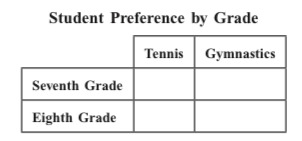Use the data from the survey to complete the table. Show or explain how you got your answers.

Create and fill in the table using the table button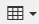Part B)